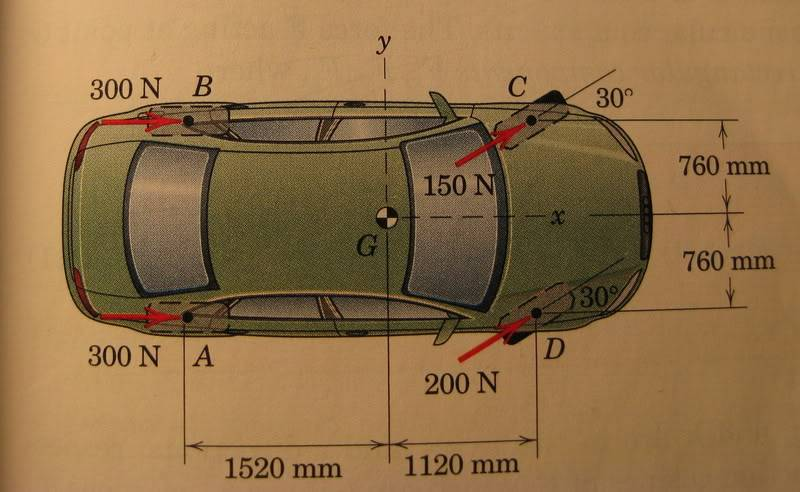Finding the x and y intercept of the line of action

Homework Statement

Determine the resultant of the system and the x- and y- intercepts of its line of action. Not that the front and rear tracks are equal (i.e., AB = CD)The Attempt at a Solution

Frx = 300 N + 300 N + 150 N*(cos30deg) + 200 N*(cos30deg) = 903.19 N

Fry = 0 + ) + 150 N*(sin30deg) +200 N*(sin30deg) = 175 N

Fr = 903.109i + 175j

||Fr|| = 919.908

angle = arctan( Fry/Frx ) = 175/903.109 N = 10.067 degrees

M FO = 760 mm*(300 N) - 760 mm*(300 N) + 1120mm*(150sin(30)) + 760 mm*(150cos(30)) - 760*(200cos(30)) + 1120 mm(sin(30)) = 51651.035 N*mm

FFrd = MO
919.909 N*d = 515651.035 N*mm
d = 56.148

I'm not sure how to find the x- and y- intercepts...can anyone lead me in the right direction please? thanks!

The x and y intercepts of what? I haven't run through the calculations to see if any mistakes were made, by the way you set the problem up seems correct.

Perhaps, if you said that G at this moment was (0,0) and treated the car as a point object initially located at G with various forces acting on it, your teacher wants to know where the car would cross the y-axis as it drove in a circle?

i think where the resultant force crosses? I'm not sure thought, i'm having trouble interpreting these questions....

I am having trouble doing the same question. I know the question is asking for the x and y intercepts of the resultant force. Where G is the point (0,0).

Shouldn't your equation for the moment be (where anti-clockwise is +ve):

M FO = 760 mm*(300 N) - 760 mm*(300 N) + 1120mm*(150sin(30)) - 760 mm*(150cos(30)) + 760*(200cos(30)) + 1120 mm(200sin(30)) = 228,908.97 N*mm

Also do you even need to calculate the magnitude of Fr? As at the y-intercept the Fy doesn't come into play, (d = Mo/Fx), as the Fy component of the resultant 'hits' point g in the centre. Vice-versa for x-intercept.

Draw a circle centre G radius d, and your resultant is acting at one of the two tangents. One spins it clockwise, the other anticlockwise. One of those tangent points will be the point x,y but which one?